## Practice Questions – Misc Aptitude – 4

1. The area of a rectangle is 221 square meter and its length is 4 meter more than its breadth. What is the measure of its breadth?

(a) 13 metre

(b) 14 metre

(c) 17 metre

(d) 19 metre

(e) None of these

2. The present age of suraj is six times the age of Vijay. Also, Vijay’s present age is 20 years less than suraj’s age. What is Suraj’s present age?

(a) 20 years

(b) 22 years

(c) 18 years

(d) 16 years

(e) None of these

3. A number when added to two-third of itself gives the value 150. What is that number?

(a) 60

(b) 75

(c) 80

(d) 90

(e) None of these

4. What will be the simple interest accrued on a sum of Rs. 4050/-at a rate of 8% p.c.p.a. in 2 years?

(a) Rs. 608

(b) Rs. 670

(c) Rs. 640

(d) Rs. 648

(e) None of these

5. Ajit has Rs. 26 more than Anuj. Anuj has R. 60 more than Ravi. If all of the three together have a total of Rs. 200, then how much amount does ajit have?

(a) Rs. 78

(b) Rs. 68

(c) Rs. 104

(d) Rs. 106

(e) None of these

6. In a test, a candidate got 38 marks less than the minimum passing marks. If the minimum passing marks are 230, then how much marks did the candidate get?

(a) 200

(b) 198

(c) 192

(d) 182

(e) None of these

7. Fifteen men can complete a piece of work in 20 days. How many men will complete the same work in 25 days?

(a) 12

(b) 13

(c) 10

(d) 8

(e) None of these

8. In how many different ways can the letters of the words SOME be arranged?

(a) 20

(b) 24

(c) 16

(d) 30

(e) None of these

1. (a) 2. (e) 3. (d) 4. (d) 5. (c) 6. (c) 7. (a) 8. (b)

Explanation

1. Let the breadth of rectangle be x metre.

Length = (x +4) metre

X (x +4) = 221 = 13 × 17

X =1 3metre

2. Let Vijay’s present age = x years

Suraj’s present age  = 6x years

6x –x = 20

5x = 20 => x = 4

Suraj’s present age = 6 × 4 = 24 years

3. Let the number be x.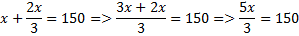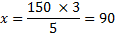4.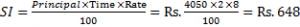5. Let the amount with ajit be Rs. x.

Amount with Anuj =Rs. (x – 26)

Amount with Ravi = Rs. (x – 86)

x + x – 26 + x – 86 = 200

3x = 200 + 112 = 312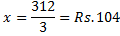6. Marks obtained by the candidate = 230 – 38 = 192

7. M1D1= M2D2

15 × 20 = M2 × 25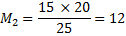8. The word SOME has 4 distinct letters

Number of arrangements = 4! = 4 × 3 × 2 × 1 = 24